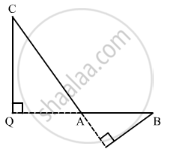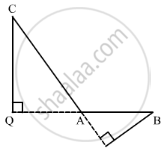Share

# In ∆Abc, ∠A is Obtuse, Pb ⊥ Ac and Qc ⊥ Ab. Prove that - CBSE Class 10 - Mathematics

ConceptApplication of Pythagoras Theorem in Acute Angle and Obtuse Angle

#### Question

In ∆ABC, ∠A is obtuse, PB ⊥ AC and QC ⊥ AB. Prove that:

(i) AB ✕ AQ = AC ✕ AP

(ii) BC2 = (AC ✕ CP + AB ✕ BQ)

#### Solution

Given: ΔABC where BAC is obtuse. PB AC and QCAB.To prove:

(i) AB × AQ = AC × AP and

(ii) BC2 = AC × CP + AB × BQ

Proof: In ΔACQ and ΔABP,

∠CAQ = BAP (Vertically opposite angles)

∠Q = P (= 90°)

∴ ΔACQ  ΔABP [AA similarity test]

rArr"CQ"/"BP"="AC"/"AB"="AQ"/"AP"            [Corresponding sides are in the same proportion]

"AC"/"AB"="AQ"/"AP"

⇒ AQ x AB = AC x AP              .....(1)In right ΔBCQ,

⇒ BC2 = CQ2 + QB2

⇒ BC2 = CQ2 + (QA + AB)2

⇒ BC2 = CQ2 + QA2 + AB2 + 2QA × AB

⇒ BC2 = AC2 + AB2 + QA × AB + QA × AB              [In right ΔACQ, CQ2 + QA2 = AC2]

⇒ BC2 = AC2 + AB2 + QA × AB + AC × AP         (Using (1))

⇒ BC2 = AC (AC + AP) + AB (AB + QA)

⇒ BC2 = AC × CP + AB × BQ

Is there an error in this question or solution?

#### Video TutorialsVIEW ALL 

Solution In ∆Abc, ∠A is Obtuse, Pb ⊥ Ac and Qc ⊥ Ab. Prove that Concept: Application of Pythagoras Theorem in Acute Angle and Obtuse Angle.
S